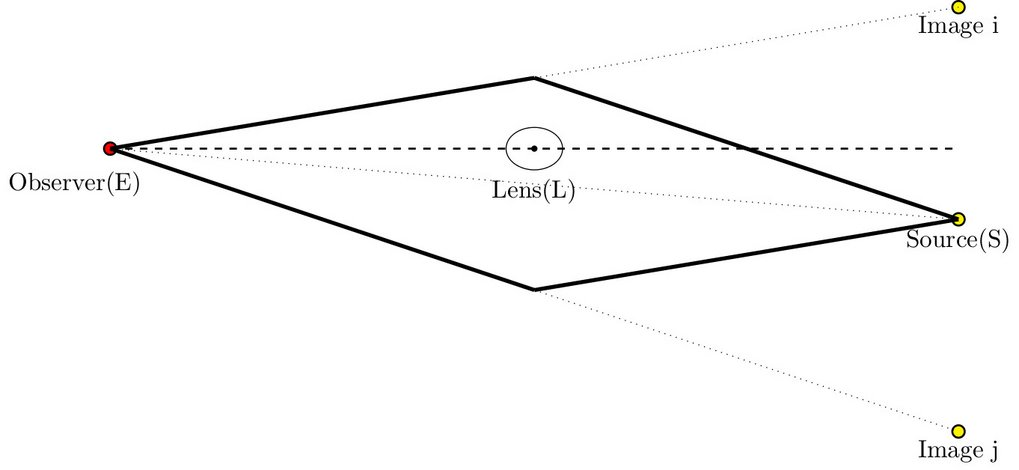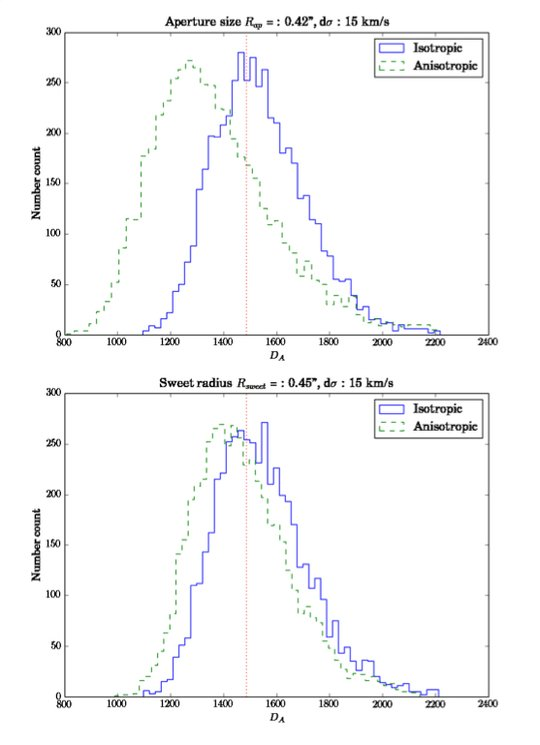# A new standard ruler: Measuring angular diameter distances using time-delay strong lenses

December 01, 2014

Since the discovery of the expansion of the universe in 1929, measuring cosmological distances has played a fundamental role in testing the cosmological models. Scientists at MPA now propose a crucially improved ruler method in addition to the well-known standard candle and standard ruler methods, which use the known luminosity or the known size of an object, respectively. For their method, they use a strong gravitational lens system with a time-varying source (e.g. a quasar) to measure the angular diameter distance to the lens. This method opens a new avenue to chart our universe and to understand the origin of the acceleration of the universe.Figure 1: Image of a strong lensing time delay system, B1608+656, taken by the Hubble Space Telescope (from Suyu et al. 2010). The centre of the lens galaxy (G1) and a satellite galaxy (G2) are surrounded by images of a background source (A, B, C and D). The ordering of the images follows the arrival time.

Observing the universe has a fundamental limitation: the 3-dimensional universe is projected onto the 2-dimensional observation plane, which makes it hard to know the 'depth' of the universe (in other words, how far an object is from us). As a consequence, astronomers rely on indirect methods to measure cosmological distances.

There are two methods to achieve this goal: One is the so-called 'standard candle' method, where the apparent luminosity of an object is compared to its intrinsic brightness that is assumed to be known. Using Type Ia supernovae as a standard candle, the method has led to the discovery of the accelerating expansion of the universe and the existence of dark energy. The other method is the 'standard ruler' method, which compares the apparent size of an object to its intrinsic size. For example, large-scale galaxy surveys allowed astronomers to use the so-called Baryonic Acoustic Oscillations as a standard ruler.

However, both existing methods have limitations. We still do not know the progenitors of Type Ia supernovae, and thus using them to infer distances may be limited by our physical understanding of supernovae. The resources required to measure Baryonic Acoustic Oscillations are large, as we need to take spectra of millions of galaxies, and a given project takes many years and tens of millions of euros (or more) to complete. Therefore, there is a strong desire to find another candle or ruler, which is well understood physically and demands less observational resources.Figure 2: Configuration of a strong gravitational lensing system. The thick solid line shows the two different paths that photons emitted from the source could take. The dotted lines show the angular positions where images and the source appear on the sky. The position of the source, however, is usually not observable as it is very close to the lens. Note that the plot is not to scale: The distance photons travel is much longer than the size of the lens or the source. Credit: Jee, Komatsu and Suyu 2014

Recently, MPA scientists have substantially improved upon a method to use strong gravitational lens systems with measured time delays to infer the distance to the lens. The method was first proposed in 2009 by Paraficz and Hjorth, but no further study has been done since then. Strong gravitational lensing happens when a massive galaxy is almost, but not exactly, aligned to a source quasar that is located behind the galaxy. The light emitted from the source quasar passes through the gravitational field of the galaxy and is slightly bent towards the galaxy, according to General Relativity.

Each photon passing through a different part of the galaxy experiences a different gravitational potential: As a result, the galaxy acts like an optical lens and the photons taking separate paths produce multiple images around the galaxy (see Figure 1). As each photon takes a different path to reach the observer, the path lengths and thus the arrival times of the photons differ, allowing the observer to measure the delay between each path as the source quasar changes its brightness in time (see schematic in Figure 2). The time difference between images is called the time delay.

The physics behind the new ruler method is simple. The measured time delay is proportional to the mass of the galaxy. The measured velocity dispersion of the galaxy gives the gravitational potential of the lens. Combining both measurements yields the physical size of the lens. Then, by comparing the physical size of the lens that has been calculated and the angular size of the lens that has been observed, we can obtain the distance to the lens.Figure 3: Distribution of angular diameter distances calculated from (top) the simulated aperture-averaged velocity dispersion of the lens system B1608+656, and (bottom) the velocity dispersion measured at the sweet-spot radius (see Figure 1). In both plots, the blue solid line shows the distribution when the input model is isotropic, whereas the green dashed line is the distribution when the simulated velocity dispersion is anisotropic. In the upper case, the distance can be determined to an accuracy of about 15%. If the radial profile of the velocity dispersion is taken into account (using the "sweet spot", see text), as the bottom panel shows, the uncertainty decreases to 12%. Credit: Jee, Komatsu and Suyu 2014

The angular diameter distance to the lens galaxy measured in this way can be expressed in terms of several observables such as the time delay, velocity dispersion, lens redshift, and image positions. It also depends on the steepness of the mass profile of the lens, which can be determined by analysing the shape and brightness distributions of the lens and images.

One big advantage of this new method, which was found by scientists at MPA, is that the effect of the external mass that lies along the line-of-sight between the observer and the source, which is called the 'external convergence', cancels out. In other words, the angular diameter distance does not change under the presence of external mass that causes extra bending of light rays. In principle, the external convergence acts like another lens added to the lens system, and adds uncertainty to the traditional cosmological measurements using strong lenses. A good analogy exists in the classical optics: It is impossible to tell the difference between a single lens system with a given focal length, and a multiple lens system consisting of lenses with different focal lengths which collectively form a system with the same effective focal length as that of the single lens. Likewise, gravitational lens systems with a single lens and multiple lenses cannot be distinguished by observables.

However, in our case the resulting angular diameter distance does not depend on the external convergence, since the time delay and the velocity dispersion are both determined solely by the properties of the lens. Applying this method to one of the existing lens systems, B1608+656 (Figure 1), the researchers find that the distance can be measured with 15% accuracy (Figure 3).

This new method requires precise estimation of the mass and the potential of the system from data. Challenges lie both in the observation and the modelling of the lens: Assuming that the lens galaxy (which is a massive elliptical galaxy in most cases) has reached dynamical equilibrium, the random motion of matter particles inside the galaxy counteracts the gravitational force so that the galaxy neither collapses nor expands. This is measured by the velocity dispersion of stars with respect to the centre of the galaxy. The Jeans equation relates the radial component of the velocity dispersion to the gravitational potential; however, it is impossible to observe just the radial component of the velocity dispersion. The measurement is done using the Doppler shift of stellar light, which means that only the line-of-sight component of the velocity dispersion is measurable. The fact that only the luminous tracers (such as stars) can be measured results in the observation of projected, luminosity weighted velocity dispersion. When the velocity dispersion is anisotropic, the situation becomes even more complicated. Moreover, the measured velocity dispersion is usually a quantity that is averaged over an aperture of size few tenth of arc seconds. In the end, it is not easy to relate the value we observe to the potential itself.

To overcome this complication, we use spatially resolved spectroscopy of the lens galaxy to obtain the radial profile of the velocity dispersion. Then, we use a radius at which the scatter between different anisotropic profiles is minimised, the "sweet spot", which was derived previously by other researchers at MPA (Churazov et al.). With this method, the uncertainty from the anisotropic velocity dispersion is minimised and the accuracy in the determination of the angular diameter distance improves to 12%.

This study demonstrates that, using a strong lens galaxy with measured time delays, the angular diameter distance can be measured accurately, providing a powerful, new way to chart our universe.

Inh Jee, Eiichiro Komatsu and Sherry H. Suyu(ASIAA)

## Other Interesting Articles

Go to Editor View